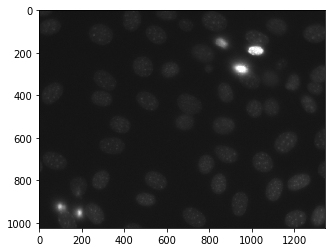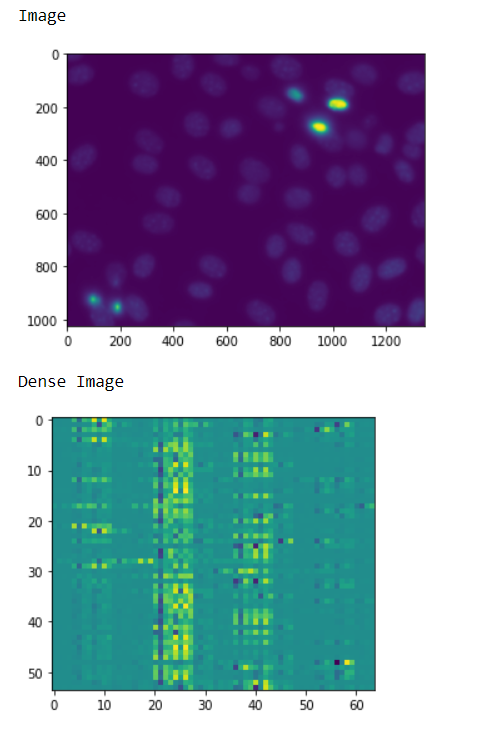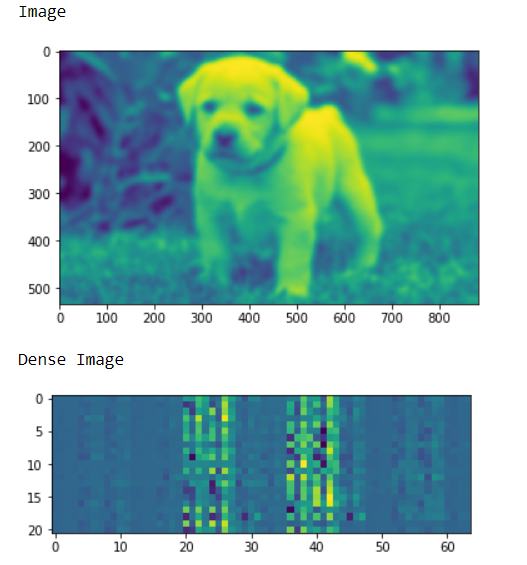# Mahotas – Getting SURF Dense Points

In this article we will see how we can get the speeded up robust dense feature of image in mahotas. In computer vision, speeded up robust features (SURF) is a patented local feature detector and descriptor. It can be used for tasks such as object recognition, image registration, classification, or 3D reconstruction. It is partly inspired by the scale-invariant feature transform (SIFT) descriptor. For this we are going to use the fluorescent microscopy image from a nuclear segmentation benchmark. We can get the image with the help of command given below

`mahotas.demos.nuclear_image()`

Below is the nuclear_imageIn order to do this we will use `surf.dense` method

Syntax : surf.surf(img, spacing)

Argument : It takes image object and integer as argument

Return : It returns numpy.ndarray i.e descriptors at dense points

Example 1 :

 `# importing various libraries ` `import` `mahotas ` `import` `mahotas.demos ` `import` `mahotas as mh ` `import` `numpy as np ` `from` `pylab ``import` `imshow, show ` `from` `mahotas.features ``import` `surf ` ` `  `# loading nuclear image ` `nuclear ``=` `mahotas.demos.nuclear_image() ` ` `  `# filtering iamge ` `nuclear ``=` `nuclear[:, :, ``0``] ` ` `  `# adding gaussian filter ` `nuclear ``=` `mahotas.gaussian_filter(nuclear, ``4``) ` ` `  `# showing iamge ` `print``(``"Image"``) ` `imshow(nuclear) ` `show() ` ` `  ` `  `# getting Speeded-Up Robust dense points ` `dense_img ``=` `surf.dense(nuclear, ``120``) ` ` `  `# shwoing image ` `print``(``"Dense Image"``) ` `imshow(dense_img) ` `show() `

Output :Example 2 :

 `# importing required libraries ` `import` `numpy as np ` `import` `mahotas ` `from` `pylab ``import` `imshow, show ` `from` `mahotas.features ``import` `surf ` `  `  `# loading iamge ` `img ``=` `mahotas.imread(``'dog_image.png'``) ` ` `  `    `  `# filtering the imagwe ` `img ``=` `img[:, :, ``0``] ` `     `  `# setting gaussian filter ` `gaussian ``=` `mahotas.gaussian_filter(img, ``5``) ` `  `  `# showing iamge ` `print``(``"Image"``) ` `imshow(gaussian) ` `show() ` ` `  ` `  `# getting Speeded-Up Robust dense points ` `dense_img ``=` `surf.dense(gaussian, ``80``) ` ` `  `# shwoing image ` `print``(``"Dense Image"``) ` `imshow(dense_img) ` `show() `

Output :My Personal Notes arrow_drop_upCheck out this Author's contributed articles.

If you like GeeksforGeeks and would like to contribute, you can also write an article using contribute.geeksforgeeks.org or mail your article to contribute@geeksforgeeks.org. See your article appearing on the GeeksforGeeks main page and help other Geeks.

Please Improve this article if you find anything incorrect by clicking on the "Improve Article" button below.

Article Tags :

Be the First to upvote.

Please write to us at contribute@geeksforgeeks.org to report any issue with the above content.